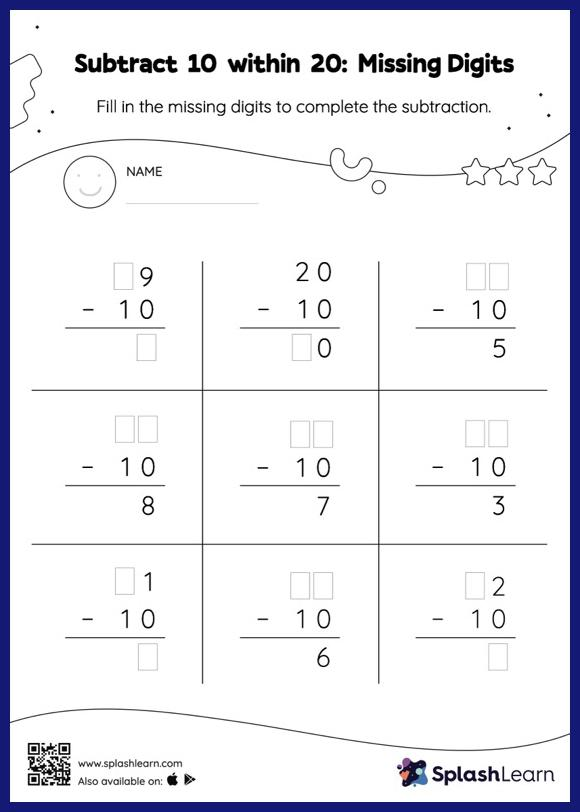# Subtract 10 within 20: Missing Digits Worksheet

Home > Subtract 10 within 20: Missing DigitsIf the missing number is a minuend or subtrahend, students use the count back strategy or the relationship between addition and subtraction to find the missing number. And if the missing number is the difference of the subtraction sentence, they subtract the numbers to get the sum. This worksheet, subtract 10 within 20, provides plenty of practice with this concept. In each problem, the numbers are laid out in the vertical format. In this format, pair of digits in each successive place are tackled one by one. This helps students follow a structured approach.